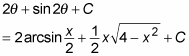##### Calculus II For DummiesWhen the function you’re integrating includes a term of the form (a2bx2)n, draw your trig substitution triangle for the sine case. For example, suppose that you want to evaluate the following integral: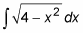This is a sine case, because a constant minus a multiple of x2 is being raised to a power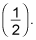Here’s how you use trig substitution to handle the job:

1. Draw the trig substitution triangle for the correct case.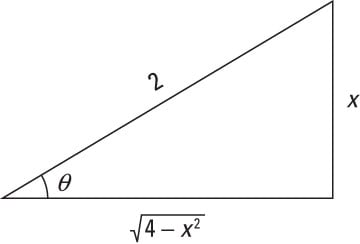This figure shows you how to fill in the triangle for the sine case. Notice that the radical goes on the adjacent side of the triangle. Then, to fill in the other two sides of the triangle, you use the square roots of the two terms inside the radical — that is, 2 and x. Place 2 on the hypotenuse and x on the opposite side.

You can check to make sure that this placement is correct by using the Pythagorean theorem: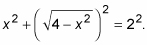2. Identify the separate pieces of the integral (including dx) that you need to express in terms of theta.

In this case, the function contains two separate pieces that contain x: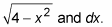3. Express these pieces in terms of trig functions of theta.

This is the real work of trig substitution, but when your triangle is set up properly, this work becomes a lot easier. In the sine case, all trig functions should be sines and cosines.

To represent the radical portion as a trig function of theta, first build a fraction using the radical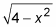as the numerator and the constant 2 as the denominator. Then set this fraction equal to the appropriate trig function: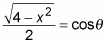Because the numerator is the adjacent side of the triangle and the denominator is the hypotenuse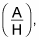this fraction is equal to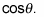Now a little algebra gets the radical alone on one side of the equation: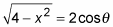Next, you want to express dx as a trig function of theta. To do so, build another fraction with the variable x in the numerator and the constant 2 in the denominator. Then set this fraction equal to the correct trig function: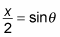This time, the numerator is the opposite side of the triangle and the denominator is the hypotenuse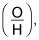so this fraction is equal to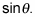Now solve for x and then differentiate: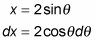4. Rewrite the integral in terms of theta and evaluate it: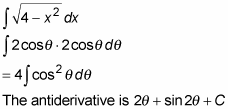5. To change those two theta terms into x terms, reuse the following equation: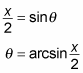So here’s a substitution that gives you an answer: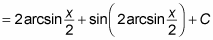This answer is perfectly valid so, technically speaking, you can stop here. However, some professors frown upon the nesting of trig and inverse trig functions, so they’ll prefer a simplified version of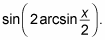To find this, start by applying the double-angle sine formula to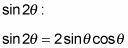Now use your trig substitution triangle to substitute values for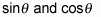in terms of x: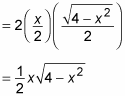To finish, substitute this expression for that problematic second term to get your final answer in a simplified form: